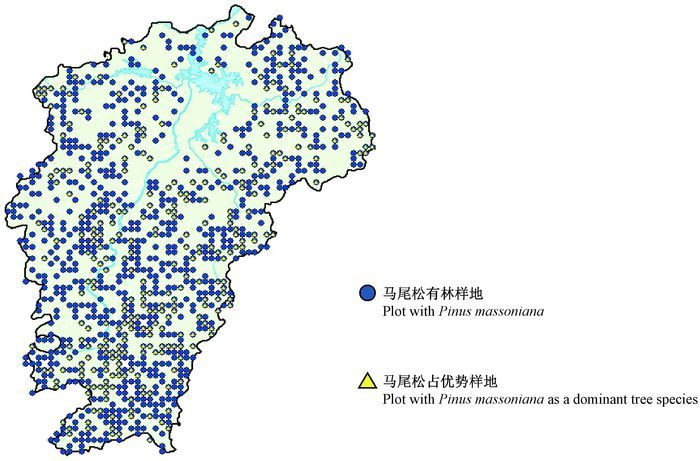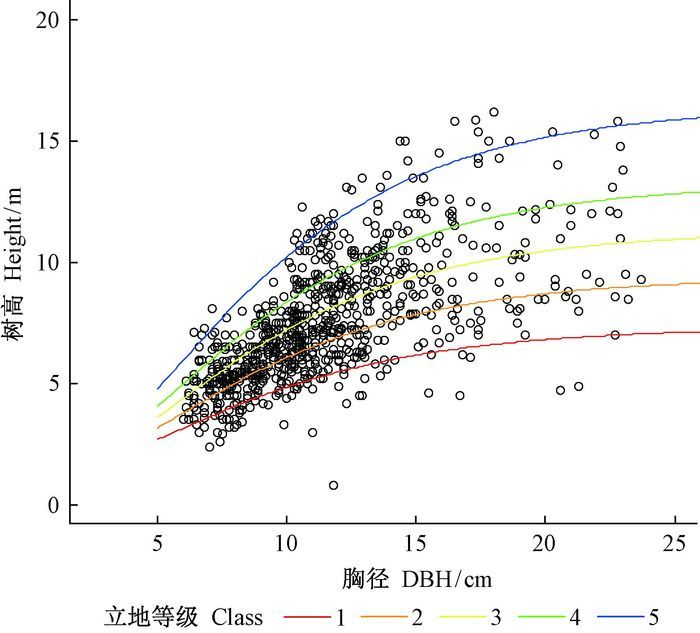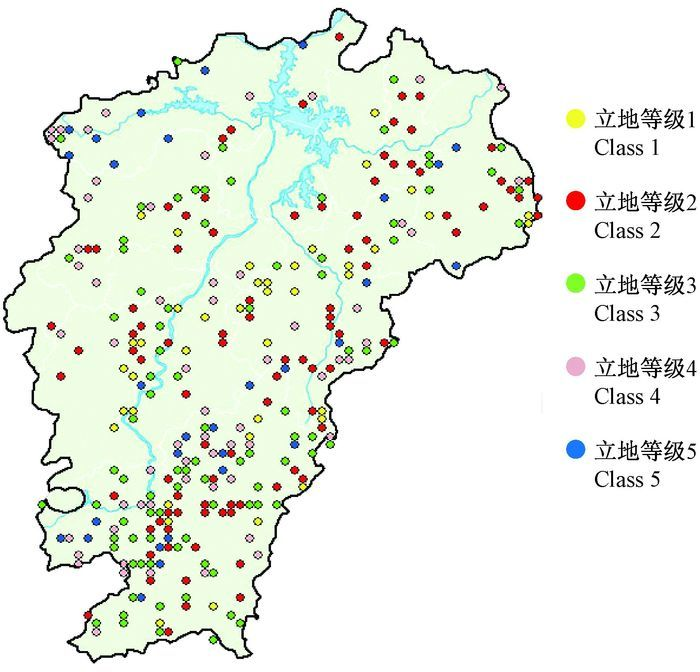﻿ 江西省不同立地等级的马尾松林生物量估计和不确定性度量
 林业科学2017, Vol. 53Issue (8): 81-93PDF
DOI: 10.11707/j.1001-7488.20170810
0

#### 文章信息

Zhao Han, Lei Yuancai, Fu Liyong

Biomass and Uncertainty Estimates of Pinus massoniana Forest for Different Site Classes in Jiangxi Province

Scientia Silvae Sinicae, 2017, 53(8): 81-93.
DOI: 10.11707/j.1001-7488.20170810

### 作者相关文章

Biomass and Uncertainty Estimates of Pinus massoniana Forest for Different Site Classes in Jiangxi Province
Zhao Han, Lei Yuancai, Fu Liyong
Research Institute of Forest Resource Information Techniques, CAF Beijing 100091
Abstract: 【Objective】 To obtain the regional tree aboveground biomass and its uncertainty estimate on different site quality and choose the optimizational model for biomass estimation, this study presented a novel method to obtain more accurate estimates of forest biomass in the forest productivity estimation.【Method】 The regional site quality classification in Pinus massoniana forests of Jiangxi Province was determined using the dominant tree height (H)-diameter at breast height (D) model. The aboveground biomass density and its root mean square error (RMSE) in each site class were estimated by the Monte Carolmethod based on the three allometric biomass models including (1) gi=aDib+ε, (2) gi=a(Di2Hi)b+ε, and (3) gi=aDibHic+ε, where gi is the individual biomass of the ith sample tree, Di and Hi are the diameter at breast height (DBH) and tree height for the ith sample tree, respectively; a, b and c are model parameters; ε is the error term.【Result】 1) The coefficient of determination (R2) obtained from the three biomass equations are more than 0.95, which indicated that the three equations have good fitting abilities. Among the candidate models, Model (3) showed the best performance. 2) The dominant H-D model showed a good fitting ability with R2=0.907, mean error (ME)=0.001, mean absolute error (MAE)=0.559, and RMSE=0.027. Plots classified by site quality were distributed to all the regions of Jiangxi Province and the sample plots in the same site level were relatively concentrated. 3) The simulation studies using Monte Carlo method were achieved stability by 10 000 times repeats. Aboveground biomass estimates calculating by the same individual tree biomass equation increased with increasing level of site. The middle site class level (the third level) represents the mean level of the regional site conditions and has similar biomass estimate with the whole region. Under the same site class, the order of mean aboveground biomass estimate values of the three models was the following:equation (1) > equation (3) > equation (2) and the order of both RMSE and relative RMSE estimates values was the following:equation (2) < equation (3) < equation (1).【Conclusion】 1) The equation (2) is better than equation (3) and then the equation (1) by comparing the relative RMSEs of the mean biomass density estimate. 2) The more similar the site quality is to the mean site quality level, the smaller the relative RMSE of the aboveground biomass density will be. 3) This study put forward a method to estimate the regional tree biomass and uncertainty in different site quality by combining the H-D model and the Monte Carlo simulation, and provides a probability and reference to accurately estimate the site productivity and biomass under different site quality.
Key words: site classification    allometric model    biomass estimates    uncertainty estimates    Monte Carlo simulation

1 研究区概况与数据 1.1 研究区概况图 1 研究区域和样地设置 Fig.1 Study region and sample plots set
1.2 数据收集与处理2 研究方法

2.1 单木地上生物量异速生长模型的建立

 ${{g}_{i}}=aD_{i}^{b}+\varepsilon ;$ (1)
 ${{g}_{i}}=a{{(D_{i}^{2}{{H}_{i}})}^{b}}+\varepsilon ;$ (2)
 ${{g}_{i}}=aD_{i}^{b}H_{i}^{c}+\varepsilon 。$ (3)

2.2 SD样木的树高估计

 $h=1.3+a{{(1-{{e}^{-bd}})}^{c}}+e。$ (4)

2.3 基于优势木树高分级的立地分级

1) 将SCD中的胸径数据按照2 cm划分径阶，计算每个径阶下树高最大值和最小值之差，除以要划分的等级数求得级差，在每个径阶内将树高按下式分级：

 $\text{class}=\left\{ \begin{array}{*{35}{l}} k\ \text{if}\ \text{min}{{H}^{D}}+\left( k-1 \right)H_{\text{class}}^{D}\le {{h}_{ij}}＜\text{min}{{H}^{D}}+kH_{\text{class}}^{D} \\ k=1,2,\cdots ,m-1 \\ m\ \text{if}\ \text{min}{{H}^{D}}+\left( k-1 \right)H_{\text{class}}^{D}\le {{h}_{ij}}\le \text{max}{{H}^{D}} \\ k=m \\ \end{array} \right.$

2) 将每个径阶中相同等级的样木数据合并。为了方便起见，使用一个哑变量矩阵来表示每株样木的等级，表示方法如下：

 $\underset{1\times m}{\mathop{\text{Des}{{H}_{ij}}}}\,=\left\{ \begin{array}{*{35}{l}} 0 & k=\text{class} \\ 1 & k\ne \text{calss} \\ \end{array} \right.k=1,2,\cdots ,m。$

 ${{h}_{ij}}=1.3+\underset{1\times m}{\mathop{\text{Des}{{H}_{ij}}}}\,\underset{m\times 1}{\mathop{a}}\,\text{ }{{(1-{{e}^{-b{{d}_{ij}}}})}^{c}}+{{e}_{ij}}。$ (5)

3) 重复步骤2，直到各样木分级不再发生变化，得到样木水平的树高分级和分级拟合的曲线。

4) 由于样地面积未超过0.1 hm2，因此规定样地内所有样木均属于同一立地等级。计算样地上每株样木在每条曲线下的预测值，按照残差平方和最小的原则对样地进行重新分级，不断重复这一步骤直到样地分级结果不再发生变化，使得样地上所有样木都获得相同的等级，获得样地水平的树高分级结果。

2.4 蒙特卡洛法模拟生物量均值及误差估计

1) 基于BMD，根据3种建模公式预测第i株样木地上生物量的无偏估计${{{\hat{g}}}_{i}}$

 $\begin{matrix} {{{\hat{g}}}_{i}}={{\beta }_{0}}D_{i}^{{{\beta }_{1}}}; \\ {{{\hat{g}}}_{i}}={{\beta }_{0}}{{(D_{i}^{2}{{H}_{i}})}^{{{\beta }_{1}}}}; \\ {{{\hat{g}}}_{i}}={{\beta }_{0}}D_{i}^{{{\beta }_{1}}}H_{i}^{{{\beta }_{2}}}。\\ \end{matrix}$

2) 假设残差服从正态分布ε~N(0, σ2)，标准差σ满足：式(1) E(ln $\hat{\sigma }$)=γ1+γ2· ln D；式(2) E(ln $\hat{\sigma }$)=γ1+γ2· ln D+γ3 ln H；式(3) E(ln $\hat{\sigma }$)=γ1+γ2· ln D+γ3 ln H (McRoberts et al., 2001)。其中，E(ln $\hat{\sigma }$)表示ln $\hat{\sigma }$的均值，$\hat{\sigma }$σ的样本估计值，γ1γ2γ3为参数。随机生成一组服从

 ${{\varepsilon }_{\beta }}\tilde{\ }N[0,~\text{exp}2({{\gamma }_{1}}+{{\gamma }_{2}}\cdot \text{ln}D)],$ (6)
 ${{\varepsilon }_{\beta }}\tilde{\ }N[0,~\text{exp}2({{\gamma }_{1}}+{{\gamma }_{2}}\cdot \text{ln}D+{{\gamma }_{3}}\text{ln}H)],~$ (7)
 ${{\varepsilon }_{\beta }}\tilde{\ }N[0,~\text{exp}2({{\gamma }_{1}}+{{\gamma }_{2}}\cdot \text{ln}D+{{\gamma }_{3}}\text{ln}H)]$ (8)

3) 将生物量估计值${{{\hat{g}}}_{i}}$与新的残差εβ合并得到$g_{i}^{\prime }$，作为新的单木地上生物量，与生物量建模数据中的胸径因子拟合，得到新的单木地上生物量估计模型：

 $\begin{matrix} g_{i}^{\prime }={{{\hat{g}}}_{i}}+{{\varepsilon }_{\beta }}={{\alpha }_{0}}\cdot D_{i}^{{{\alpha }_{1}}}; \\ g_{i}^{\prime }={{{\hat{g}}}_{i}}+{{\varepsilon }_{\beta }}={{\alpha }_{0}}\cdot {{\left( {{D}^{2}}_{i}{{H}_{i}} \right)}^{{{\alpha }_{1}}}}; \\ g_{i}^{\prime }={{{\hat{g}}}_{i}}+{{\varepsilon }_{\beta }}={{\alpha }_{0}}\cdot D_{i}^{{{\alpha }_{1}}}\cdot H_{i}^{{{\alpha }_{2}}} 。\\ \end{matrix}$

4) 用新的参数和生物量预测数据中的胸径因子来估计不同立地质量等级k下第j块样地第t株样木的地上生物量gjt，合计每个立地等级下所有样地的生物量，计算该立地等级下的生物量均值Gk并推算误差Var(Gk)：

 $\begin{matrix} {{{\bar{G}}}_{k}}=\frac{1}{n}\sum\limits_{j=1}^{n}{\sum\limits_{t=1}^{f}{{{g}_{jt}}}}; \\ \text{Var}({{{\bar{G}}}_{k}})=\frac{1}{n\left( n-1 \right)}\sum\limits_{j=1}^{n}{{{(\sum\limits_{t=1}^{f}{{{g}_{jt}}-{{{\bar{G}}}_{k}}})}^{2}}}。\\ \end{matrix}$

5) 重复2、3、4步骤，直至结果趋于平稳。推算每个立地等级k下的生物量在m次模拟后的均值μkm及误差RMSEkm和相对误差Relative RMSE km

 $\begin{matrix} \mu _{k}^{m}=\frac{1}{m}\sum\limits_{x=1}^{m}{{{({{{\bar{G}}}_{k}})}_{x}}}; \\ \text{RMSE}_{k}^{m}=\sqrt{\left( 1+\frac{1}{m} \right){{U}_{1}}+{{U}_{2}}}; \\ \text{Relative}\ \text{RMSE}_{k}^{m}=\frac{\text{RMSE}_{k}^{m}}{\mu _{k}^{m}}\cdot 100\%。\\ \end{matrix}$

2.5 模型评价

 $\begin{matrix} \text{ME}=\sum\limits_{i=1}^{n}{(y_{i}^{\prime }-{{y}_{i}})/n}; \\ \text{MAE}=\sum\limits_{i=1}^{n}{|y_{i}^{\prime }-{{y}_{i}}|/n;} \\ \text{RMSE}=\sqrt{\sum\limits_{i=1}^{n}{{{(y_{i}^{\prime }-{{y}_{i}})}^{2}}}/\left( n-p \right);} \\ {{R}^{2}}=1-\frac{\sum\limits_{i=1}^{n}{{{(y_{i}^{\prime }-{{y}_{i}})}^{2}}}}{\sum\limits_{i=1}^{n}{{{\left( {{y}_{i}}-{{{\bar{y}}}_{i}} \right)}^{2}}}}; \\ R_{\text{adj}}^{2}=1+\left( {{R}^{2}}-1 \right)\frac{n-1}{n-k-1}。\\ \end{matrix}$

3 结果与分析 3.1 3种单木地上生物量模型比较3.2 SD样木树高估计3.3 优势木树高分级模型与立地分级图 2 优势木树高-胸径拟合曲线 Fig.2 Dominant trees height-diameter classification curve图 3 马尾松优势林样地立地分级示意 Fig.3 Site quality classes diagram of sample plots with Pinus massoniana as dominant tree species
3.3 生物量均值与不确定性度量

 $\begin{matrix} \varepsilon \tilde{\ }N[0,~\text{exp}2(-3.877+2.281\cdot \text{ln}D)]; \\ \varepsilon \tilde{\ }N[0,~\text{exp}2(-3.147+1.738\cdot \text{ln}D+0.198\cdot \text{ln}H)]; \\ \varepsilon \tilde{\ }N[0,~\text{exp}2(-3.432+2.614\cdot \text{ln}D+0.681\cdot \text{ln}H)]。\\ \end{matrix}$4 讨论

5 结论

3种单木地上生物量模型形式下的马尾松地上生物量估计结果相比，加入树高变量的式(2) 和式(3) 生物量估计误差的绝对值和相对值均好于式(1)，加入树高变量的单木生物量模型能够提高估计精度。在胸径和树高2种变量同时存在时，拥有2个参数的式(2) 较有3个参数的式(3) 获得了更好的估计效果。

 [] 傅煜, 雷渊才, 曾伟生. 2015. 单木生物量模型估计区域尺度生物量的不确定性. 生态学报, 35(23): 7738–7747. ( Fu Y, Lei Y C, Zeng W S. 2015. Uncertainty analysis forregional-level above-ground biomass estimates based on individual tree biomass model. Acta Ecologica Sinica, 35(23): 7738–7747. [in Chinese] ) [] 李海奎, 雷渊才. 2010. 中国森林植被生物量与碳储量评估. 北京, 中国林业出版社. ( Li H K, Lei Y C. 2010. Estimation and evaluation of forest biomass carbon storage in China. Beijing, China Forestry Publishing House. [in Chinese] ) [] 曾伟生. 2014. 3种异速生长方程对生物量建模的对比分析. 中南林业调查规划, 33(1): 1–3. ( Zeng W S. 2014. Comparison of three allometric equations for biomass modeling. Central south forest inventory and planning, 33(1): 1–3. [in Chinese] ) [] Berrill J P, O'Hara K L. 2013. Estimating site productivity in irregular stand structures by indexing the basal area or volume increment of the dominant species. Canadian Journal of Forest Research, 44(1): 92–100. [] Chave J, Condit R, Aguilar S, et al. 2004. Error propagation and scaling for tropical forest biomass estimates. Philosophical Transactions of the Royal Society of London B:Biological Sciences, 359(1443): 409–420. DOI:10.1098/rstb.2003.1425 [] Cohen R, Kaino J, Okello J A, et al. 2013. Propagating uncertainty to estmates of above-ground biomass for Kenyan mangroves:a scaling procedure from tree to landscape level. Forest Ecology and Management, 310: 968–982. DOI:10.1016/j.foreco.2013.09.047 [] Cole T G, Ewel J J. 2006. Allometric equations for four valuable tropical tree species. Forest Ecology and Management, 229(1): 351–360. [] Fang J, Chen A, Peng C, et al. 2001. Changes in forest biomass carbon storage in China between 1949 and 1998. Science, 292(5525): 2320–2322. DOI:10.1126/science.1058629 [] Gargaglione V, Peri P L, Rubio G. 2010. Allometric relations for biomass partitioning of Nothofagus antarctica trees of different crown classes over a site quality gradient. Forest Ecology and Management, 259(6): 1118–1126. DOI:10.1016/j.foreco.2009.12.025 [] Huang S, Titus S J. 1993. An index of site productivity foruneven-aged or mixed-species stands. Canadian Journal of Forest Research, 23(3): 558–562. DOI:10.1139/x93-074 [] Ketterings Q M, Coe R, van Noordwijk M, et al. 2001. Reducing uncertainty in the use of allometric biomass equations for predicting above-ground tree biomass in mixed secondary forests. Forest Ecology and Management, 146(1): 199–209. [] Li H, Zhao P. 2013. Improving the accuracy oftree-level aboveground biomass equations with height classification at a large regional scale. Forest Ecology and Management, 289: 153–163. DOI:10.1016/j.foreco.2012.10.002 [] McRoberts R E. 1996. Estimating variation in field crew estimates of site index. Canadian journal of forest research, 26(4): 560–565. DOI:10.1139/x26-064 [] McRoberts R E, Westfall J A. 2014. Effects of uncertainty in model predictions of individual tree volume on large area volume estimates. Forest Science, 60(1): 34–42. DOI:10.5849/forsci.12-141 [] Pérez-Cruzado C, Rodríguez-Soalleiro R. 2011. Improvement in accuracy of aboveground biomass estimation in Eucalyptus nitens plantations:effect of bole sampling intensity and explanatory variables. Forest Ecology and Management, 261(11): 2016–2028. DOI:10.1016/j.foreco.2011.02.028 [] Peri P L, Gargaglione V, Pastur G M, et al. 2010. Carbon accumulation along a stand development sequence of Nothofagus antarctica forests across a gradient in site quality in Southern Patagonia. Forest Ecology and Management, 260(2): 229–237. DOI:10.1016/j.foreco.2010.04.027 [] Petersson H, Holm S, Ståhl G, et al. 2012. Individual tree biomass equations or biomass expansion factors for assessment of carbon stock changes in living biomass-a comparative study. Forest Ecology and Management, 270: 78–84. DOI:10.1016/j.foreco.2012.01.004 [] Rock J. 2007. Suitability of published biomass equations for aspen in Central Europe-results from a case study. Biomass and Bioenergy, 31(5): 299–307. DOI:10.1016/j.biombioe.2007.01.003 [] Satoo T, Madgwick H A I. 1982. Biomass//Madgwick H A I. Forest biomass. Netherlands:Springer, 46-89. [] Skovsgaard J P, Vanclay J K. 2008. Forest site productivity:a review of the evolution of dendrometric concepts for even-aged stands. Forestry, 81(1): 13–31. DOI:10.1093/forestry/cpm041 [] Ståhl G, Holm S, Gregoire T G, et al. 2011. Model-based inference for biomass estimation in a LiDAR sample survey in Hedmark County, Norway. Canadian Journal of Forest Research, 41(1): 96–107. DOI:10.1139/X10-161 [] Wang C. 2006. Biomass allometric equations for 10 co-occurring tree species in Chinese temperate forests. Forest Ecology and Management, 222(1): 9–16.